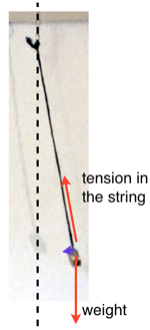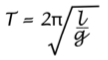Simple Harmonic Motion (SHM) of a pendulum

Although commonly used in the teaching of simple harmonic motion a swinging pendulum does not perfectly fit the conditions for SHM. However, if we are careful, a swinging pendulum moves in very nearly simple harmonic motion. The angle of swing must be small and the mass must be large compared to the mass of the string/wire.The restoring force (that is the force towards the centre of the swing) is provided by the vector sum of the two forces acting on the bob. These are the tension in the string and the weight. Because the size of the restoring force depends on the mass and the acceleration is inversely proportional to the mass, the effect of the mass cancels out. The time of the swing is independent of the mass.

In school experiments the time of swing is often used to calculate the acceleration of gravity / gravitational field strength, g. To calculate the value of g we have to rearrange the equation for the time period of a pendulum:Squaring both sides we get:

T2=4π2 l/g  then if we rearrange this for g we get  g = 4π2 l/ T2

In an experiment to determine the acceleration due to gravity g we have to measure the length of the pendulum and the time period. The length is from the pivot point to the centre of the mass.

One swing of the pendulum is from any point (usually one end) and then across and back to the same point going in the same direction. The time periods are likely to be fairly short and hard to measure accurately. To avoid this error we measure a number, perhaps ten or twenty and then divide to get the value of one swing.

To finally determine the acceleration due to gravity g we aim to  reduce systematic errors in the calculation. First we make measurements of the time period of a number of lengths of the pendulum. Then we plot a graph l against T2 . This is a way of checking because all the points should be on or close to a straight line.